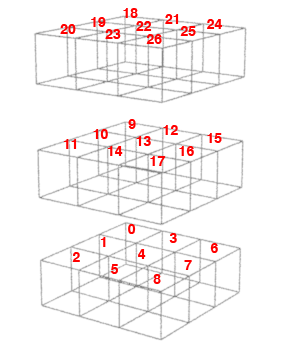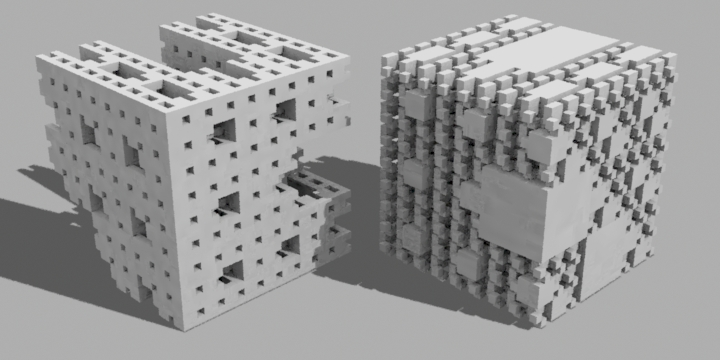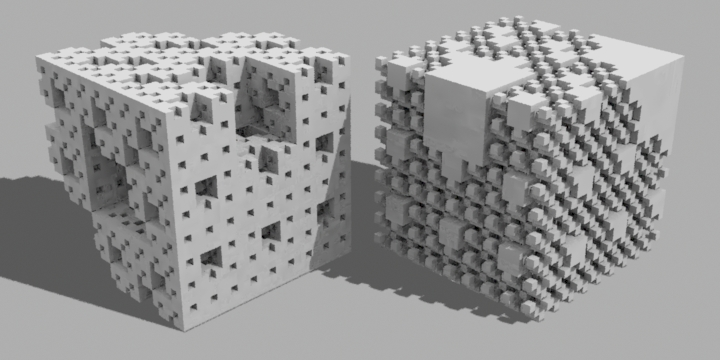### Menger SpongePython

`Source Code:`
menger3d.py
ri_utils.py

#### Introduction

A typical cubic Menger sponge is shown in figure 1. It is a fractal object created by recursively replacing a cube with a grid of 27 sub-cubes of which 7 are removed. Figure 2 shows four objects where the depth of recursion is 0, 1, 2 and 3.Level 0: 1 cube
Level 1: 20 cubes
Level 2: 400 cubes
Level 3: 8000 cubes
Figure 2

The cube numbering system used by menger3d.py is shown in figure 3. A classic menger sponge normally removes the cubes with indices (from top to bottom) 22,16,14,13,12,10 and 4.Figure 3

#### Not-A-Menger & Holes

However, more interesting visual results can be achieved by removing an arbitary sequence of cubes at each level of recursion. Some examples are shown below. Each image shows the modified Menger sponge on the left and the "holes" on the right. The images were rendered with Pixar's PRMan.Figure 4